content

# The phenomenon of retarded potentials

Dynamics of interactions.
Absence of a general principle of relativity.
The phenomenon of longitudinal vibrations of moving bodies.
The causal basis of wave quantum mechanics and nuclear energy.

Nikolay NOSKOV
Translated from Russian by Jury SARYCHEV
English text edited by Robert FRITZIUS

Ye shall make you no idols...

Leviticus 26, 1

It is necessary to become indifferent and to not be fixed on the question of whether there is an advantage in truth, or whether it will be fatal to you... It is necessary, as that is inherent for a strong person, to prefer questions, which today nobody dares to pose. Courage is necessary to enter forbidding areas; as it is necessary for an inclination to live in a labyrinth. And a seven-fold experience of loneliness is needed. And new ears for new music are needed. And new eyes, capable of discerning new horizons, are needed. New consciences to capture truth, heretofore unspoken...

Friedrich NIETZSCHE. "Antichrist"

Since Descartes and Newton, researchers of physical phenomena came to the inference that the interactions between bodies are transmitted through a medium which exists between them. This medium, which we shall name after Huygens as ether, should have all the properties of matter, in the sense that we understand them: mass as a measure of inertness, hardness, elasticity, plasticity, density, movement, etc... In this case, as the guarantee of the correctness of the physical laws, such as: the laws of the conservation of energy, impulse, momentum and others, we should accept the invariance of Euclidean space, mass and time in the manner that they were postulated by Newton in "The Beginnings..." .

Mass, as the carrier of energy, can transfer and transmit it from one point of space to another. That is the main condition for transmission of an interaction potential with a finite velocity depending on the properties of the transmitting medium and on the mechanism, with the help of which this interaction occurs. The mechanisms of interaction can be divided into four kinds:
1) the transfer of energy occurs together with a transfer of the medium;
2) waves in the medium;
3) transmission of pressure through the medium;
4) aerohydrodynamical.

For a long time it has been accepted that the last three mechanisms for the defined medium have the same velocity of transfer. So in air the transmission of sound waves and of pressure occurs with the same speed. Carrying out this analogy, it is possible to reach the inference that the velocity of gravitational, electromagnetic and nuclear interactions, considering, that their mechanisms are carried out through the same medium – the ether, occurs with the velocity of waves in this medium, i.e., with the velocity of light. C.F. Gauss in 1835, P. Gerber in 1898, H. Poincaré in 1902 and many others came to such conclusions. But as usual the researchers went no further than these conclusions. As it turns out, the most importance starts from these conclusions.

At first Gauss mused on the consequences of the existence of a finite speed for interactions. In 1835 he deduced a law for the dynamics of electromagnetic particle-particle interactions depending on the relative velocity of the interacting particles, where Coulomb electrostatics was generalized by the velocity of the interactions :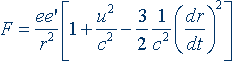, where: (1)

ee'/r2 – Coulomb's law in the agreed on system of units;

(3/2c2) (dr/dt)2 – the coefficient of retarded potential;

u – relative velocity between electrons;

c – velocity of interactions, equal to the velocity of light;

dr/dt – absolute velocity between the bodies along a line connecting them.

And though this law appears not to be absolutely correct, the logical approach to its inference, its idea, was important. Gauss's law was the result of modeling the process of interactions. The reasoning is simple and logical, and the results are repeatable and verifiable.

Gauss reasoned approximately in the following manner. If the velocity of propagation of an interaction potential is finite, (this is not generally doubted by anyone now) it arrives at a moving body with some delay, since from the moment of its departure from the first body, the second one has moved to another position. In this case the law of interaction for motionless bodies provides the arrival of a potential with a slightly different value. The greater the absolute velocity between bodies, the more the potential delay. When the velocity of the bodies equals the velocity of the interaction, a complete delay occurs (the potential from the first body can not reach the second) and the interaction between the bodies is absent, its force is equal to zero.

Gauss came to the conclusion that the law of interactions should depend on its mechanism. But so far as it is impossible to reveal this law at present, probably there are overlooked ways, which would provide a check on the heuristically inferred laws from observations, experiments and empirical laws. First of all, Ampere's law regarding the interaction of two current carrying wires became such an empirical law.

Gauss died not having had time to publish his discovery. But he had time to mail a letter in Leipzig to his younger colleague and friend W. Weber, with whom he had worked a long time in Gottingen. In the letter he stated his reasonings on this matter and the law of electrodynamics (the law of potential delay), that he had deduced. Before publishing Gauss' letter in the collection of his works in 1867, Weber had deduced and published in 1846 his own law of particle–particle electrodynamics :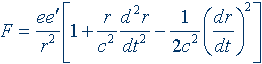, where: (2)

(1/2c2) (dr/dt)2 – delay factor,

c – conversion factor from electrostatic to electrodynamic systems of units.

Concerning this law it is necessary to make the following remarks. If the first derivative of distance with respect to time is equal to zero, the potential delay is absent. If the second derivative is equal to zero, there is no radiation. There is also a third possibility. The first derivative is not equal to zero (there is a potential delay), and the second derivative is equal to zero (the velocity along a line connecting bodies is constant). This corresponds to elliptical motion (it is a question of whether an electron should radiate in orbital motion).

Weber deduced the particle-particle law from Ampere's law for the interaction of two current carrying wires and produced it as a formalism, not explaining its causal substantiation. Instead of the velocity of interaction the conversion factor from the electrostatic to the electrodynamics system of units appears in it. After that Weber together with R. Kohlrausch showed experimentally, that the value of this coefficient is equal to the velocity of light (As naturally it should be, according to Gauss!). And all have understood that light is electromagnetic oscillations. Weber's law attracted the researchers' attention. So classical electrodynamics was born.

Two great physicists, H. Helmholtz and J.C. Maxwell, understood the formalism of Weber as a law of a long-range action (where the coefficient is not the velocity of interaction) and criticized it severely. Helmholtz saw in Weber's law an infringement of the law of the conservation of energy. And it is a fair assessment, regarding potential delay, one which else nobody guessed nor understood, which in itself means a non-instantaneous realization of the spent energy, but rather there is some retardation. Maxwell recounted: "The energy of interaction has already left one body, but has not reached the other!" Helmholtz's error is very well "elucidated" by him in the foreword to the book by H. Hertz "Principles of Mechanics Stated in a New Connection" . He wrote: "The laws of electrodynamics were derived in Germany from a hypothesis of Weber, who tried to reduce the explanation of electrical and magnetic phenomena to a certain modification of the Newtonian supposition about forces, operating directly and rectilinearly across a distance... In the hypothesis of Weber the supposition was made, that the propagation of forces, in infinite space, occurs instantly with infinite velocity (Emphasis added. – N.N.)... Similar hypotheses were put forward by F. Neumann, his son K. Neumann, Riemann, Grossmann, and later by Clausius. Clear inferences did not at all follow from this mixed assembly of hypotheses. To make them, it was necessary to resort to complicated calculations, to the decomposition of separate forces into their variously directed components and so forth. So the area of electrodynamics turned into an impassable desert during that time. The facts based on observations, and the consequences from the rather doubtful theories – were pell-mell intertwined."

We see now, that Helmholtz was wrong himself. But he was a known and rather influential scientist during that time and his opinion played a major role. His convincing rehabilitation of Maxwell's laws in his "Treatise on Electricity and Magnetism"  has remained unnoticed by subsequent generations of physicists. Following a review of Gauss's reasonings he devoted a whole chapter to the laws of Gauss and Weber, having shown, that both laws are equally derived from Ampere's law, and that they are laws of the short range picture, and that Weber's law is subject to the law of the conservation of energy.

However an even more negative role in the rejection of the laws of potential delay was strangely enough played by another very famous and influential scientist-physicist, H. Lorentz. This was because at one time he had continued the researches on electrodynamics and, having united the approaches of Clausius and Maxwell, obtained his famous law of electrodynamics for a charged moving particle interacting with electric and magnetic fields :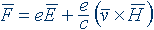. (3)

This expression is known as "the Lorentz Relation" and it comes from the electron theory, which was created by Lorentz in 1892. He determined the correlation between the forces of interaction on a charged particle (an electron) by electrical and magnetic fields, depending on the electron's velocity. He should have explained the abnormal deviations in the movement of electrons in a transverse magnetic field in the experiments of J.J. Thomson and W. Kaufmann and if his expression did not conform to experiment, its adjustment would be required. Really, considering it now from the viewpoint of the phenomenon of potential retardation, we see, that though the force of interaction here depends on the velocity of the electron. Potential retardation, which would reduce it in compliance with the ratio v2/c2, as it occurs in the laws of Clausius, is absent. It induces the thought that Lorentz vaguely imagined the ideas of Gauss. Yet, R. Feynman  showed that the values of E and H in formula (3) should be represented using the law of retarded potential, having indicated, that the principle of operation of current generators, and a feature of the behavior of light is contained in it also. In short, the retarded potential touches all phenomena of electricity and magnetism." Nevertheless, Lorentz did not go any further with these inferences and did not try to apply them to the experiments of Kaufmann.

J.J. Thomson was the first to notice the anomalous deviations in the movement of an electron in a transverse magnetic field from the laws of classical mechanics in 1881. He explained results of his experiments by using the laws of classical mechanics. It did not occur to him to apply electrodynamics to explain the results of his experiments, because the reasonings of Gauss were unknown to him. The law of Weber was a vague formalism, and the electron theory of Lorentz had only been in existence for 11 years. He came to this inference as though obvious: that with the increase of velocity of electrons their mass increases.

When in 1902-1903 Kaufmann ,  repeated the experiments of J.J. Thomson, he did not reflect on the reasons for the anomalous deviations in the movement of electrons at all. His task was finding an empirical law. Here, again, there was a surprising coincidence: the law of assumed change of mass of an electron approximately coincided with Lorentz's factor, which he applied as a hypothesis of the shortening of the longitudinal linear sizes to explain the "null" results of the Michelson-Morley experiments.

In the period from 1881 to 1904 the most important law in the development of physics – causality was infringed by researchers J.J. Thomson, W. Kaufmann, and Lorentz by the invention of a certain "electromagnetic mass," the substance of which was confirmed by nothing. It was impossible to make an inference about what parameter was variable and why without the elucidation of causality. Besides, Lorentz twice incorrectly interpreted the results of the experiments. The results of the Michelson and Morley experiments were not zero, and those of Kaufmann were not absolutely satisfactorily attributed to the Lorentz factor.

The coincidence of the law of assumed increase of mass of an electron, according to Lorentz's factor, played a major role in the introduction of the special theory of relativity. Lorentz was already considering the elucidation of it. And after he, with much blood and sweat, managed to win the victory over Fresnel's hypothesis about the partial drag of ether by bodies, the "gift" of the increase of mass of electrons simply fell into his hands. In his 1904 article "The Electromagnetic Phenomena in a System Moving with any Velocity, Less than the Velocity of Light"  Lorentz used all the results of the experiments of Kaufmann and showed their good correlation with the hypothesis about the increase of mass of an electron by application of the formula bearing his name. In the same article he finally generated the idea of a general principle of relativity. The creation and the ground of errors was completed. H. Poincaré (after some indecision) and A. Einstein (without any and without critical analysis) transferred these errors into their works. Just this fact especially plainly establishes the priority of Lorentz on the introduction of a general principle of relativity. Let's notice, however, that Poincaré completely recognized the priority of Lorentz. Poincare wrote about it and spoke repeatedly of it, when he discussed Lorentz' works in his articles (1898 to 1905).

Lorentz's priority is especially convincingly expressed in Poincaré's main work  which was issued practically simultaneously with the article of Einstein "On the Electrodynamics of Moving Bodies" . In the beginning of the work "On the Dynamics of an Electron" he writes: "According to the theory of Lorentz two equal segments – by definition – will be such two segments, through which light passes in the same time. Maybe, it is enough to refuse this definition only, that the theory of Lorentz was completely omitted, as it happened with the system of Ptolemy after the innovation of Copernicus". It is all in the citation. There is the recognition of priority. The postulate about the constancy of the velocity of light (in the sense of the relativistic formula of the addition of velocities) from the theory of Lorentz follows. The postulate came to be attributed to Einstein in consequence, and it was entered insufficiently correctly, causing doubts as to its justice. Those with ears, let them hear!

This discussion about priority, lasting already more than 90 years, looks rather strange on the background of this statement of Poincaré. However the close reader of Einstein's works should notice, that he did not make a procedure of introducing a general principle of relativity, but postulated it as an accomplished fact. The purpose of his works was to show the consequences of the introduction of a general principle of relativity. As they were destructive (all of the invariants of classical mechanics fell disastrously to the principle of the equivalence of mass and energy, the paradox of the twins and so on), the work of Einstein for this reason at once became the center of attention. Here again the group of physicists played an unseemly role, elevating Einstein and his work on a pedestal.

* * *

It will be shown below, that the law of potential retardation leads to the inference that as the velocity of an electron approaches the velocity of interactions (which is equal to velocity of light), the force of the interactions will be equal to:

 Emax = mec2 · f 2/2, where: (4)

me – mass of electron;

c – velocity of interaction, equal to velocity of light;

f = vlin.max. / vph – factor, dependent on the law of potential retardation.

The classical law of energy is not infringed in formula (4). The energy has a finite value.

This is compared to the relativistic formula

 E = mc2, where: (5)

m – relativistic mass, equal to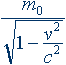;

c – velocity of light (notice, there is no velocity of interactions here already!);

In formula (5) the electron mass, and consequently its energy, tends to infinity at the approach of the velocity of the electron to c. Being accelerated to a velocity close to c (with the help of a cyclotron) in penetrating a target the electron would destroy the target and cyclotron as well. However such does not occur, as greater energy, than in (4) can not be obtained. The electron mass (in formula (4)) does not change its value but the energy increases because the frequency of the longitudinal oscillations increases (see below). It is expressed by the factor f. The factor f is not the Lorentz factor so far as it has a definite value at any velocity of interaction, as the Lorentz factor approaches zero as v → c and mass approaches infinity.

Considering the above discussion, it is possible to make the inference that one of the main bases for the introduction of a general principle of relativity drops out, namely: the new coordinate transformations in the invariant form of the Lorentz group are not valid for dynamics.

And though nobody up till now has chosen to notice, the second postulate about the constancy of the velocity of light (in the sense of the relativistic formula for the addition of velocities) for a long time has failed also. A direct consequence of this postulate is the assertion about the impossibility for any electromagnetic experiments (in particular, the experiments of Michelson–Morley) to detect movement of an inertial system, considered here to be the Earth. This was the main argument for the existence of a general principle of relativity.

From the very beginning this postulate was presented with a distortion of the results of the Michelson–Morley experiments , . The movement of the Earth with respect to ether with a speed of 3 to 7.5 km/s was the result of those experiments. Though this value was much less expected, it was not a null result and it tended to confirm the Fresnel hypothesis about the partial drag of ether, rather than showing its absence.

However experiments by D.C. Miller  showed that with an increase of distance from the surface of the Earth the velocity of the ether increases. He came to the conclusion that with respect to the structure of Solar system the Earth has total movement toward the North with a speed more than 200 km/s (it can be 300-400 km/s). He made it on the basis of experiments and according to Fresnel's hypothesis about the partial dragging of ether.

The inference of Miller was excellently confirmed in 1958 by American physicists , who made measurements of the anisotropy of background radiation in space, which relativists consider as relic radiation. The Earth, participating in the motion of the Solar system, moves with respect to the background radiation with a speed ≈400 km/s toward the North.

Detection of movement of inertial systems, with the help of electromagnetic experiments, which is what the measurements of anisotropy of background radiation do, refute the relativistic formula for addition of velocities and, therefore, the postulate about the constancy of the velocity of light relative to the observer.

Thus, all bases for the introduction of a general principle of relativity have disappeared. It does not exist in nature. Its introduction by Lorentz, Poincaré and Einstein has cast physics for a whole century into an abyss of mathematical formalism, beginning from the general theory of relativity (GTR) of Grossmann – Einstein, finishing with the relativistic theory of gravitation (RTG) of Logunov – Mestverishvily, etc.

The researchers of our planet have gotten into a trap, an infinite labyrinth from which there is no exit at present nor can there be. Year after year, from grounds of dissatisfaction and of critical approaches to GTR 2...3, new mathematical formalisms are appearing as if correcting and improving it. A set of new directions, such as many-dimensional spaces, superstrings and others have arisen. The methodology of the GTR has had an influence on electrodynamics, quantum mechanics, and on the physics of elementary particles, by having retarded their development.

* * *

The phenomenon of retarded potential, revealed by Gauss, and which is a fundamental law of nature covers all sorts of interactions. And first of all it concerns gravitation.

It is natural, that the researchers, who have understood it, have immediately begun to make attempts to find the law of graviodynamics. As for electromagnetic interactions H.C. Örsted, F. Arago, Ampere and Faraday determined the separate properties of electrodynamics by means of experiments. That allowed checking up its correctness. As for gravitation there was a unique observational fact discovered by U.J.J.Le Verrier in 1859 – the anomalous offset of the Mercury's perihelion, equal to 41" per century.

W. Ritz, F. Zöllner, F. Tisserand, C. Seegers, G. Holzmüller, W. Scheibner, M. Levy, Ljenar, Schwarzschild, and J.C. Maxwell are not the complete list of the researchers who tried to discover the law of graviodynamics. They represented physical schools of three states of Europe: England, France and Germany. The overwhelming majority of scientists, as well as those who discovered the laws of electrodynamics, among which were Gauss, Weber, Clausius, F. Neumann, C. Neumann, Riemann, Grossmann and others, were from Germany.

The long time attempts to create a law of gravitation with a retarded potential that take into account the mechanism of interactions and give the correct value of anomalous advances of the perihelia of planets, came to an unsuccessful end. After several thwarted attempts to obtain the law of graviodynamics Maxwell wrote, "Being unable to understand, how the medium can have such a property, I can not advance further in this direction in searches into the reasons of gravitation".

But the researcher who bypassed the difficulties of modeling the mechanism of interactions has been found. He was Paul Gerber, a teacher from Stargard. He reasoned as follows. Because the interaction is transmitted through the medium from point to point, the velocity of an interaction potential depends on the properties of the medium and has a terminal value.

The terminal velocity of interaction entails the lag of potential for bodies moving relatively to each other and interacting with each other. This lag of potential is distributed across the distance between bodies, and its value, in such cases, is inversely proportional to velocity of a body.

Having taken Newtonian potential:

 V0 = m1m2 / r , (6)

having substituted instead of the distance r, which should be traversed by a retarded potential from m1 to m2: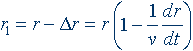, where: (7)

v – velocity of propagation (of the interaction);

and also having entered the proportionality of delay in units of time multiplied by velocity, which is equal to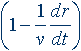, (8)

he obtained this expression for potential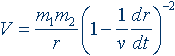. (9)

Having substituted it in the standard equation of Lagrange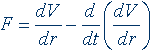, (10)

he obtained a law of graviodynamics from three factors similar to those for Weber's law of electrodynamics: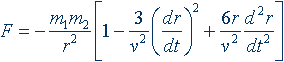, (11)

which is remarkable in that if it is accepted that the velocity of interaction v is equal to the velocity of light c, the predicted advances of perihelia of planets are equal to their observables.

The article by Gerber under the name "Space and Timing of the Propagation of Gravitation"  was published in Z. Math. Phys., 43, p. 93-104 in 1898, i.e., 17 years prior to GTR, whose appearance was connected with an explanation of the anomalous advancement of the perihelion of Mercury.

Now, when it becomes clear, that a general principle of relativity does not exist and that GTR also appeared without foundations, there is a question: why its predictions do not contradict observable phenomena? The point is, and I wrote about it in the article "The General Principle of Relativity Does Not Exist" , that the Lorentz factor, being a key in the GTR, for velocities of bodies under v = 0.85 c, correlates with the factor of retarded potential. Lorentz was convinced of that for Kaufmann's experiments. Those who attentively read Lorentz's article, should notice, that for v > 0.85 c the divergence from Lorentz's factor becomes somewhat greater. If in this experiment these velocities were to come nearer to c, the discrepancy would become significant, as the application of Lorentz's factor would lead to an increase of mass and energy up to infinity, while in experiments the energy of an electron can not exceed values defined by equation (4). The transverse magnetic field simply would cease to have an influence on the movement of the electron, and the electron would move rectilinearly.

All the above-stated reasons against a general principle of relativity and in favor of the phenomenon of retarded potential have been met with objections. They are made by the virtue of the conservatism of scientists, educated by schools on relativism in which there is a refutation of common sense, which itself is based on the strictness of logical laws, on causality and understanding – the main laws in the development of physics. It is frequently possible to hear that since the theory of relativity agrees with the observable phenomena, it has a right to existence and so on. On this idea I would like to relate the following. Any incorrect theory or error has always retarded the development of science. And especially then, when they became dominating and generally accepted, as it was, for example, with the Ptolemaic system. However, in this case, the possibility has appeared to present new arguments for the benefit of the proof of the fidelity of the delayed potential theory.

In the textbooks of physics at schools and universities practically nothing is presented about the phenomenon of retarded potential. And what has been spoken is only in the sense of the opinion of Helmholtz regarding the "school of a long-range picture". I was lucky enough to reveal it for myself anew, irrespective of Gauss. The pressure of accepted authority had no influence over me, which has allowed me to advance in research of retarded potential even further than just writing about the laws of interaction dynamics. Soon I discerned that my attempts on writing of the laws of delay were not necessary, seeing as very remarkable physicists, such as Gauss, Weber, Clausius, Ritz, Maxwell, Gerber and others have already worked on them. But, as to the prospective discovery of the phenomenon of longitudinal oscillations of moving bodies, these researches are original. No one prior to me has done them. To let you know about that, I needed to reconsider all the developments of physics since the initial appearance of the general principle of relativity , , , , and .

* * *

Simulating the process of retarded potential on a moving test body  with the help of three variables: the velocity of the body, the force of interaction and the distance between the bodies, I have determined that the retarded potential occurs non-uniformly, in an undulatory fashion. It means, that the motion of bodies under the influence of a force (electrical or gravitational fields, atmospheric or liquid pressure differentials or others) occurs with longitudinal oscillations. The average velocity of a body driving with oscillations is a phase velocity.

The logical goal is finding out the direct or inverse proportionality of length of the oscillations for all three variables that lead to the expression: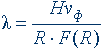, where: (12)

λ – length of oscillations;

H – factor of proportionality;

vph – phase velocity of a body;

R – distance between test and central bodies R(t);

F(R) – law of interaction.

So far as

 λ = vph / v , а (13)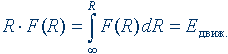, то (14)

then formula (12) will be transformed into:

 Eosc = Hvph / λ = Hv. (15)

However, the energy of oscillations of a body can be expressed as it is done in classical dynamics through vlin.max.:

 Emove = mv2lin.max. / 2, where: (16) vlin.max. = vph f (17)

– momentary linear maximal velocity of a body, where: f = vlin.max./vph – is the coefficient depending on the law of retarded potential.

Then formula (16) can be written in the form:

 Emove = mv2ph f 2 / 2 (18)

The appearance of this formula also explains why the formula of energy of classical dynamics E = mv2/2 is infringed. At small velocities, v << u, where u is the velocity of interaction, the ratio f 2/2 is practically not different from 1/2 if v approaches u then f approaches any given maximum value and formula (18) will be:

 Emove = αmeu2 , where (19)

α – the maximum value f 2/2 of the velocity of interaction.

Equating (15) and (18), because they are expressions of the same energy of motion, we shall obtain an additional formula of length of longitudinal oscillations for moving bodies: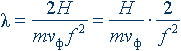, (20)

which is a modification of the de Broglie formula.

The so-called "correlation of de Broglie for wave lengths":

 λ = h / mv, where: (21)

h – Planck's constant; v – the linear velocity of the electron;

is retrieved by de Broglie heuristically and intuitively but until now there has been no causal physical explanation. However it has played an outstanding role in the occurrence of wave quantum mechanics.

The interpretation of this equation as a wave of longitudinal oscillations of moving bodies, allows us to assert, that wave quantum mechanics is solution of a system of two wave equations for a moving body (in particular, an electron in an atom). Where the first equation is for a longitudinal oscillation, the second is for a cyclic oscillation near the central body. The solution of this system is the resonance of oscillations and, as result, – steady discrete orbits.

An analysis of the Schrödinger equation, made in 1969 by two Soviet scientists A.A. Sokolov and I.M. Ternov  also confirms this inference. They have shown, that the stationary (non time-dependent) Schrödinger equation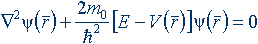(22)

is a system of three equations: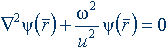, – (23)

and is the equation of a monochromatic space wave in a homogeneous medium, where: λ = 2πu / ω, and then: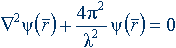; (24)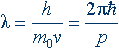– (25)

is the expression of de Broglie's wavelength:

 p2 / 2m0 + V(r) = E = const (26)

Equation  is the law of conservation of energy for an orbit.

The joint solution of (24) and (26) represents, as it were, the expression of a temporal cyclic frequency. [(24), (26)] and (25) is a resonance between the cyclic and de Broglie's frequencies. "As it were" is used because the cyclic frequency is inherent only for a rigid body in motion, while Schrödinger described the oscillations of a medium. Thus, we see that in a set of Schrödinger equations the equations for oscillations of the medium and the bodies are wrongfully combined.

We find the equation of cyclic oscillations from the Newtonian equality of forces on an orbit:

 mv2 / R = F(R), where: (27)

mv2 / R – centrifugal force;

F(R) – centripetal force (the law of interaction).

From (27) we find the cyclic frequency: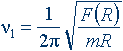; (28)

and from (20) – the frequency of longitudinal oscillations: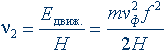. (29)

And then the universal equation of wave quantum mechanics for any interaction, depending on the law F(R), will be a condition of resonance:

 v1 = v2 . (30)

In summary it is necessary to elucidate two important consequences of the prospective discovery of the phenomenon of longitudinal oscillations of moving bodies.

The first consequence concerns the proportionality factor H in equations (12), (15) and (20). It is obvious, that H is not only invariant, since it depends on a sort of interaction [see equation (12)] but also it is not constant at all, as it depends on the value of the mass of the body. Thus, "Planck's constant" is only a local constant for electromagnetic interactions and only for masses equal to the mass of an electron.

The second important consequence is the causal explanation of so-called nuclear energy. We have already shown above, that the energy of a moving particle (body) is expressed by formula (18). It includes the energy of longitudinal oscillations, which are non-linearly dependent on velocity. The smaller the orbit of a particle in atom or nucleus the greater its velocity and the greater its energy differs from the energy defined by Newtonian dynamics. At cessation of orbital movement (at the decay of a nucleus) and the decrease in velocity of a particle down to the average velocity of Brownian motion of the surrounding medium the released energy will be equal to:

 ΔE = Hv1 – Hv2, (31)

that is confirmed by the energy of radiation by the jumping of an electron from one orbit to another.

* * *

The researches conducted as stated above, and also the observation of an enclosing environment lead to an output: the phenomenon of longitudinal oscillations of moving bodies, as a result of non-uniform retarded potentials, is one of the main processes in all natural phenomena and literally fills and penetrates the world enclosing us. It is the basis of the structure and stability of nuclei, atoms, and planetary as well as stellar systems. It is the main reason of the occurrence of sound (in particular, the voices of people, animals, and birds; the sound of wind instruments etc.), electromagnetic oscillations and light, whirlwinds, pulsations of flowing water, and gusts of wind. It, at last, explains elliptical orbital motion, for which a central body is at one of the foci instead of at the geometrical center of the ellipse. Moreover, the ellipse can not be arbitrary, so far as the lengths of the cyclic and longitudinal oscillations have different values at a resonance v1 = v2. This circumstance determines ellipticity in each concrete case.

The longitudinal oscillations of moving bodies explain all experiments on diffraction and on interference of electrons, tunnel effects and many others (from a causal foundation).

## Experiments

The inferences of this article can be confirmed by experiments with particle accelerators and cyclotrons.

The energy of accelerated particles can be defined by formula (15) Emove = Hv, where H is the constant for particles of a given defined mass, and v is the frequency of the longitudinal oscillations of the particles.

The frequency of longitudinal oscillation v can be defined from diffraction and interference patterns of the particles at reflection, or by their passage through crystal lattices. This method can lead to the creation of stationary instruments, with the help of which it will be possible almost instantly to define the energy and velocity (using formula (20)) for accelerated particles.

Similar experiments are widely enough known and were made for electrons in 1927 by Davisson and Jermer in connection with confirmation of de Broglie's observations. Further, the experiments were not only done for the diffraction of electrons, but also for other particles, i.e., atoms and molecules. "So, it was experimentally confirmed, that particles: electrons, nuclei, molecules, and neutrons – have wave properties" .

Even more, Davisson and Jermer made the very important inference: "electrons behave as a wave, the length of which depends on their velocity and which corresponds to calculations using de Broglie's formula". But the formula, having the form of "a correlation of de Broglie for wavelengths," is obtained in an offered article just from the formula of an energy E = Hv! Hence, basically, the experiments of Davisson and Jermer already are in confirmation of the conclusions of "The phenomenon..."

Thus, it was already possible to make the inference that the energy of particles depends on the frequency of their oscillations. But formula (15) was defined only for electromagnetic oscillations (light) and de Broglie waves – as "waves of probability". Besides, relativistic theory "has already defined" the dependence of the anomalous increase of energy of particles with their increase in mass according to their velocity. And it already could not be contradicted.

Now, it is enough to define the equality of energies obtained by calorimetric methods and using formula (15) with the help of diffraction, as it becomes abundantly clear, that anomalous energy of accelerated particles increases because of increases in the frequency of their velocity-induced longitudinal oscillations, and not from increases in their mass. Consequently, the theory of relativity does not exist any more.

Literature:

1. Newton, I., Philosophiae Naturalis Principia Mathematica. 1687.
2. Gauss C.F. Werke, vol. 5, Königliche Gesellschaft der Wissenschaften zu Göttingen, Göttingen, s. 616...617, 1867.
3. Weber. W., Elektrodynamische Massbestimungen über ein allgemeines Grundgesetz der elektrischen Wirkung. Abh. Begründ. K. Sächs. Wiss. (Lpz), s. 211...378, 1846.
4. Helmholtz H., The foreword to the book of. H. Hertz "Principles of Mechanics Stated in a New Connection". Acad. Sci. USSR, p. 296, 1959, in Russian.
5. Maxwell J.C., Treatise on Electricity and Magnetism. v. 2, Clarendon Press, Oxford, 1873.
6. Lorentz H.A., The Electronic Theory. Leiden, 1892.
7. Feynman R.P., Leigton R.B., Sands M., The Feynman Lectures on Physics. Mir, M., v. 3, p. 39, 1963, in Russian. [In the English version this is Volume II, Chapter 21].
8. Kaufmann. W., Phys. Zeits., 1902, b. 4, s. 105.
9. Kaufmann. W., Gott. Nachr., Math. – Phys. Klasse, 1903, s. 90.
10. Lorentz H.A., Electromagnetic Phenomena in a System Moving with Any Velocity Less Than Light. Proc Acad. Sci., Amsterdam, 1904, v. 6, p. 809.
11. Poincaré H., Sur la dynamique de l'electron. Rendiconti del Circolo Matematico di Palermo, v. 21, p. 129, 1906 (has entered in printing of July 23, 1905).
12. Einstein A., Zur Elektrodynamik bewegter Körper. Ann. d. Phys., b. 17, s. 891, 1905 (Has entered in printing of June 30, 1905).
13. Michelson A.A., The Relative Motion of the Earth and the Luminiferous Ether, Amer. J. Sci., v. 22, p. 120...129, 1881.
14. Michelson A.A., Morley E.W., On the Relative Motion of the Earth and the Luminiferous Ether, Am. J. Sci., v. 34, p. 333...345, 1887.
15. Miller D.C., The Ether Drift Experiment and the Determination of the Absolute Motion of the Earth, Revs. Modern Phys. v. 5, p. 203, 1933.
16. In: G. De Vaucoulers., A. J., 58, s. 30, 1958.
17. Gerber P., Die räumliche und zeitliche Ausbreitung der Gravitation. Z. Math. Phys., v. 43, p. 93...104, 1898.
18. Noskov N.K., The general principle of relativity does not exist. "Science of Kazakhstan" #20 (56), 1995.
19. Noskov N.K., Light, photons, velocity of light, ether and others "banalities". "Science of Kazakhstan" #5 (65), 1996.
20. Noskov N.K., Gauss, Weber, Gerber and others... "Science of Kazakhstan" #13 (73), 1996.
21. Noskov N.K., "Brilliance and poverty" of the Quantum Mechanics. "Science of Kazakhstan" #1 (85), 1997.
22. Noskov N.K., The Centenary Ethereal War. "Science of Kazakhstan" #21 (105), 1997.
23. Noskov N.K., The theories of mechanisms of interaction and hypothesis about their synthesis. "Science of Kazakhstan" #16 (124), 1998.
24. Noskov N.K., To a Problem on the Restriction of Field of Application of a Classical Mechanics. SPE "Print", Institute of high-energy physics, Academy of Sciences; Kaz. SSR, Alma-Ata, 1991.
25. Sokolov A.A., Ternov I.M. The quantum mechanics and nuclear physics. M.: Prosveshchenie, 1970, p. 39...40.
26. Semenchenko, V.K., Selected chapters of theoretical physics. M.: Prosveshchenie, 1966, p. 145...151.
content